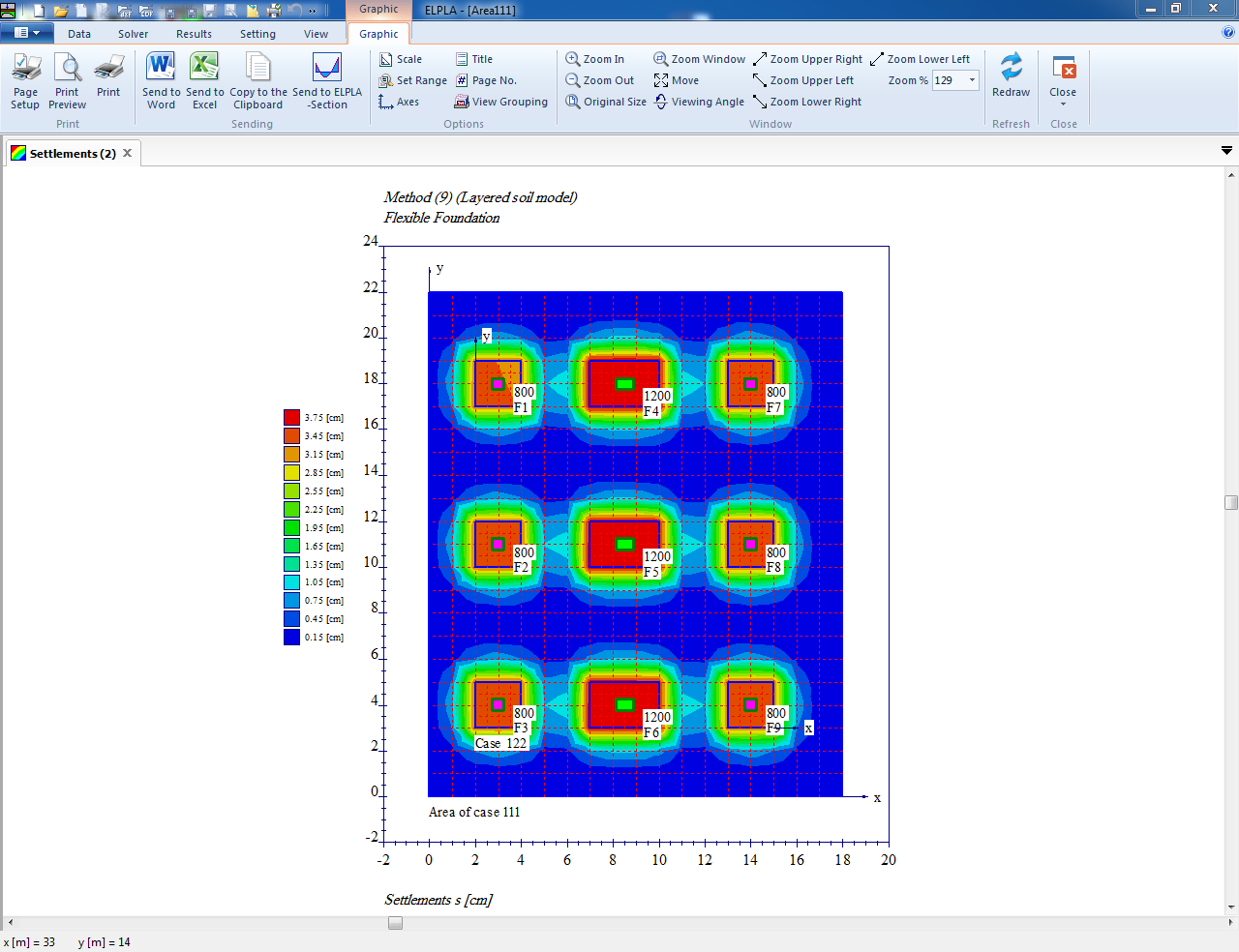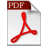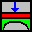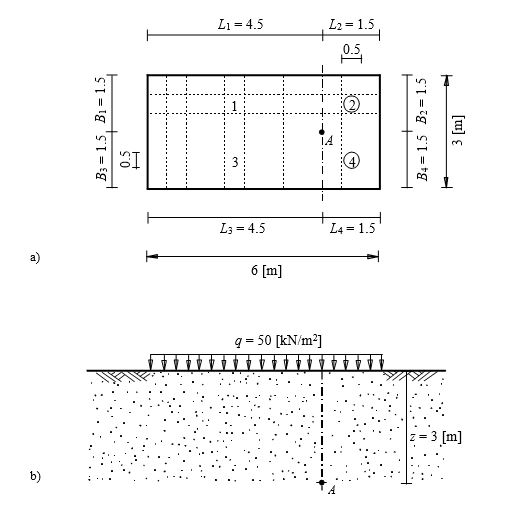أمثلة التحققThis chapter presents analysis of many foundation examples. These examples are presented in order to

• verify the mathematical models used in ELPLA by comparing ELPLA results with closed form or another published results
• illustrate how to use ELPLA for analyzing foundation by different subsoil models

The examples discussed in this chapter cover many practical problems. For each example discussed in this book, data files and some computed files are included in ELPLA software package. The file names, contents and short description of examples are listed below. Besides, a key figure of each problem that contains the main data concerning the foundation shape, loads and subsoil is also shown.

المستندات و البياناتELPLA VerificationELPLA-Data Files of Verification Examples
Example 1: Stress on soil under a rectangular loaded area
To verify the vertical stress at any point below a rectangular loaded area, the stress on soil obtained by Das (1983) (Example 6.3, page 370) using influence coefficients of Newmark (1935) are compared with that obtained by ELPLA.
A distributed load of = 50 [kN/m2] acts on a flexible rectangular area 6 [m] × 3 [m] as shown in Figure 1. It is required to determine the vertical stress at a point A, which is located at a depth of = 3 [m] below the ground surface.Example 1: Stress on soil under a rectangular loaded areaELPLA DATA-Stress on soil under a rectangular loaded area.rar